# Wave Walker DSP

## Brand New Book!

DSP for Beginners: Simple Explanations for Complex Numbers! The second edition includes a new chapter on complex sinusoids.

Folding a Polyphase Half Band Filter: Lemon Squeezer Style
October 6, 2021

#### Introduction

Folding a filter is a way to improve efficiency, and folding the weights for a polyphase filterbank improves the efficiency beyond that. The zero weights in the half band filter make for a great application of the folding and polyphase filterbank structures.

You know after you cut a lemon and try to get the juice out by hand you get to the point where you need a lemon squeezer to get every last drop? This blog is going to squeeze out every little bit of efficiency in the polyphase half band filter.The half band filter (HBF) was introduced in this blog post and transformed into a decimation by 2 polyphase filter bank (PFB) to reduce the multiplies and improve the efficiency by a factor of 2. This blog will continue by further improving the efficiency to a factor of 8 (not a typo!) by ignoring the zero weight multiples as well as folding the even-symmetric weights to reduce redundant multiplies.

Check out the other posts on half band filters:

#### Ignoring Zero Half Band Filter Weights

As discussed in this blog post about half the weights in the HBF are zero which contribute nothing to the filter output and should be ignored. An odd-length N half band filter was partitioned into branches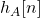and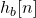, an example given in Figure 1.

The branch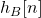is mostly zeros except for time index

(1)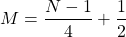and therefore can be simplified as

(2)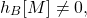(3)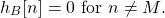The impulse response can be simplified to

(4)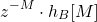which is just a delayed single weight as shown in Figure 2.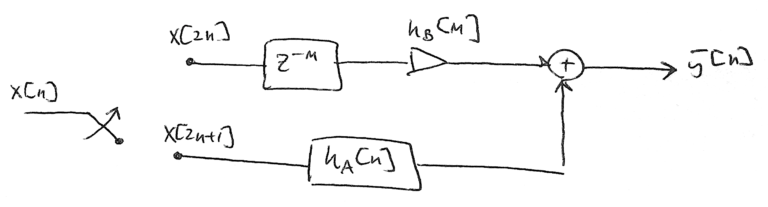Figure 2: The zero weights have been removed from the PFB implementation, leaving only a delay and a single multiply on the top branch.

The filter structure from Figure 2 is implemented in the following code:

import numpy as np

# you have to zero pad on the front to account for time-reversal in convolution!

# downsample the input
xA = x[0::2]
xB = x[1::2]

# polyphase partition of HBF
#
# the even indexing starts at one because the HBFWeights were

# the odd indexing starts at zero because the HBFWeights were

# select the non-zero weight
M = int((filterLength-1)/4 + (1/2))
hB = BWeights[M]

# implement the PFB with ignored zero weights
AOutput = np.convolve(AWeights,xA)
BOutput = np.concatenate((np.zeros(M),hB*xB,np.zeros(M-1)))

# perform summation
yPolyParallel = AOutput + BOutput

Figure 3 compares the PFB implementation of Figure 2 against the naive implementation of filtering then downsampling showing that the more efficient PBF approach produces the same exact output.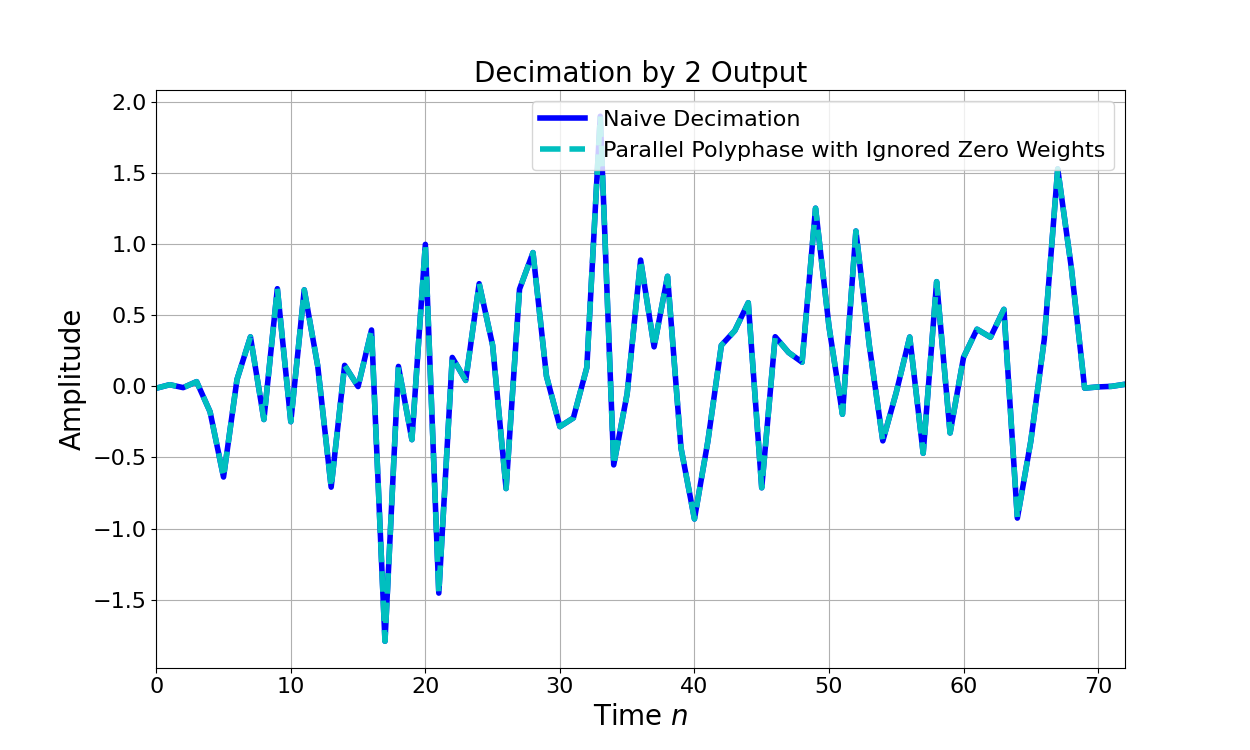Figure 3: The PFB approach with ignored zero weights produces the same output as the naive decimation implementation but with fewer computations.

#### Folded Polyphase Half Band Filter

As shown previously the weights ofare even-symmetric such that

(5)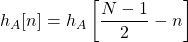for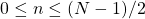. Therefore the filter weights for the branch represented bycan be folded to remove redundant multiplications [lyons2011, p.493, p.702] as shown in Figure 4.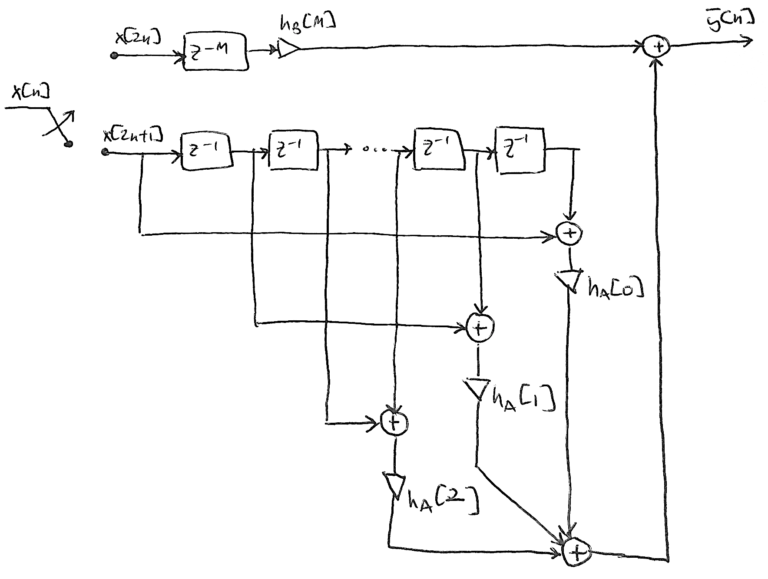Figure 4: The PFB half band filter structure after folding the even-symmetric filter weights hA[n] and ignoring the zero weights in hB[n].

Folding the even-symmetric filter weights reduces the number of multiplications by performing an extra addition of the data beforehand. As an example, without folding the filter branchwould be implemented as

(6)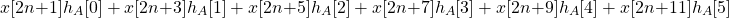which multiplies each input sample in the delay line with the corresponding filter weight. In this example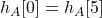,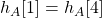and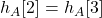. Folding and performing a pre-addition reduces the implementation to

(7)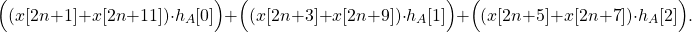The code to implement the folded PFB in Figure 4 is given below:

## implement folded branch for hA[n]# start with zeroed delay linedelayLine = np.zeros(len(AWeights))

# zero-pad the input so all input samples to the sample# buffer are filteredxAPad = np.concatenate((xA,np.zeros(len(AWeights)-1)))

# pre-allocate outputfoldedAOutput = np.zeros(len(xAPad))

for index in range(len(xAPad)):

    # add in new sample to delay line    delayLine = np.insert(delayLine,0,xAPad[index])

    # remove the last sample from the delay line    delayLine = delayLine[0:-1]

    # perform the pre-add    preAdd = delayLine[0:M] + delayLine[-1:-M-1:-1]

    # multiply weights    weightedPreAdd = preAdd*AWeights[0:M]

    # summation    foldedAOutput[index] = np.sum(weightedPreAdd)

# folded PFB outputyPolyParallelFolded = foldedAOutput + BOutput

Figure 5 gives an example of the output from the folded PFB with ignored zero weights showing it is the same output as the naive decimation approach.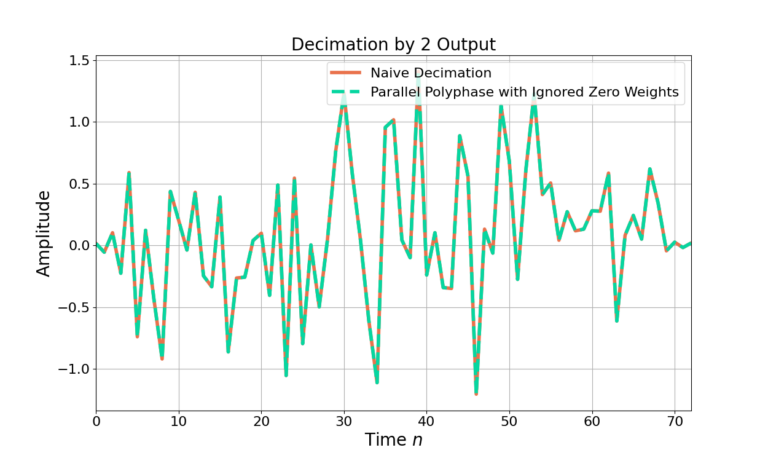Figure 5: The folded PFB with ignored zero weight implementation produces the same output as the naive decimation with even fewer multiples as in Figure 2 and Figure 3.

#### Computational Efficiency

It was shown prior that applying a PFB structure to a decimate by 2 for filter length N can reduce the amount of multiplies by 1/2. The efficiencies from ignoring the zero weights inas well as folding the even-symmetric weights inwill further reduce the number of multiplies needed to implement the filtering.

Ignoring the zero weights inleads to a single multiply for in the branch where there are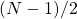multiples in thebranch in Figure 2. The number of multiplies per output sample is therefore

(8)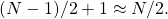As the decimation by 2 PFB structure has already been applied it therefore only takes

(9)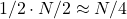multiplies per each input sample which is 4 times as efficient than the naive implementation! The benefit of the PFB approach is that the filtering can be done at the decimated output sample rate as seen here.

For example a half band filter with length N=19 the subfilter corresponding tocan be implemented with a single multiply andimplemented with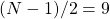for a total of 10 multiples per output sample. However due to the PFB structure the number of multiplies per input sample is 10/2 = 5.

Additional savings come from folding the even-symmetric weights in. The filter branchhas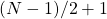weights however half of them are even-symmetric and the number of multiplies can be reduced through a pre-add as in Figure 4. The number of multiplies for an output sample for thebranch is therefore

(10)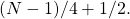Adding one multiply for thebranch to (10), the total number of multiplies per output sample for a half band folded PFB with ignored zero weights is therefore

(11)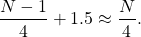For example a filter of length N=19 the filter is implemented with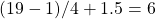multiplies.

However the PFB structure allows the filter to run at the output sample rate which is 1/2 the input sample rate, therefore the number of multiples per input sample is

(12)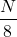which is 8 times more efficient than the naive decimator!

Improving the efficiency by a factor of 8 is a huge savings! In dB that is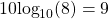dB. It’s not everyday you find a free 9 dB worth of efficiency to be gained in your system through rearranging some delays, multiplies and adds.

#### Conclusion

The efficiencies of the polyphase half band filter can be further improved by ignoring zero weights and folding redundant weights. Incorporating the filter structure in Figure 4 makes the implementation 8 times more efficient than the naive decimation approach. Half band filtering then decimating by 2 is useful in real to complex conversion and in large sample rate change through stages, both of which require efficiency since they are often dealing with the largest sample rates within a system.

I think I’ve demonstrated how to get all inefficiencies out of the HBF. If you have a way to further improve the filter structure please email me! matt@wavewalkerdsp.com

Have you seen the other posts on the half band filter?

God, the Lord, is my strength; he makes my feet like the deer’s; he makes me tread on my high places. Habakkuk 3:19

### 2 Responses

1.Joseph says:

The Homer reference was chefs kiss ?

1.WaveWalkerDSP says:

I knew you would like it!

You can check out Joe Gaeddert’s work at https://liquidsdr.org and get his Liquid DSP software on github at https://github.com/jgaeddert/liquid-dsp

This website participates in the Amazon Associates program. As an Amazon Associate I earn from qualifying purchases.